# TFA Discrete Gabor Transform VI

LabVIEW 2014 Advanced Signal Processing Toolkit Help

Edition Date: June 2014

Part Number: 372656C-01

»View Product InfoDownload Help (Windows Only)

Owning Palette: Time Frequency Transform VIs

Decomposes signal as a linear combination of Gabor elementary functions, which are complex sinusoids with a window envelope. You can use the TFA Discrete Gabor Expansion VI to reconstruct the time-domain signal. Wire data to the signal input to determine the polymorphic instance to use or manually select the instance.

Use the pull-down menu to select an instance of this VI.

 Select an instance TFA Discrete Gabor Transform (Waveform)TFA Discrete Gabor Transform (Real)TFA Discrete Gabor Transform (Complex)TFA Discrete Gabor Transform (2D)

## TFA Discrete Gabor Transform (Waveform)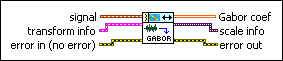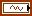signal specifies the input signal.transform info specifies the analysis window to use for the Gabor transform. You can use the Dual Window Express VI to design the analysis window.analysis window specifies the window to use to compute the Gabor coefficients. analysis window is the dual window of the synthesis window, which is the envelope of the Gabor elementary functions. The size of analysis window must be divided evenly by dM (time steps) and N (frequency bins).dM (time steps) specifies the time shift, in samples, between elementary functions. The time sampling interval of the signal in the time-frequency domain is dM (time steps)/fs, where fs is the sampling rate of the signal. National Instruments recommends that you set dM (time steps) to a power of 2.N (frequency bins) specifies the number of frequencies of the Gabor elementary functions. The frequency sampling interval of the signal in the time-frequency plane is fs/N (frequency bins), where fs is the sampling rate of the signal. N (frequency bins) must be a power of 2. For stable reconstruction, N (frequency bins) must be greater than or equal to dM (time steps). The ratio between N (frequency bins) and dM (time steps) is called the oversampling rate.error in describes error conditions that occur before this node runs. This input provides standard error in functionality.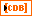Gabor coef returns the linear time-frequency representation of the signal. Gabor coef contains the weights of the Gabor elementary functions. The element in the ith row and the jth column is the weight of the Gabor elementary function whose time center and frequency center equal i×dt and j×df, respectively, where dt is the time interval between every two contiguous rows of Gabor coef, and df is the frequency interval between every two contiguous columns of Gabor coef. You can use the TFA Coefficients Indicator to display the magnitude of Gabor coef on an intensity graph. You can save the time-dependent 2D array to a text file for use in another software environment. The resulting text file contains only Z values and does not retain the time axis information or the frequency axis information. You can use the TFA Get Time and Freq Scale Info VI to compute the time scale information and the frequency scale information of the time-frequency representation.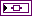scale info returns the time scale and the frequency scale information of the time-frequency representation, including the time offset, the time interval between every two contiguous rows, the frequency offset, and the frequency interval between every two contiguous columns of Gabor coef. Use the TFA Get Time and Freq Scale Info VI to return detailed information about the time scale and the frequency scale.error out contains error information. This output provides standard error out functionality.

## TFA Discrete Gabor Transform (Real)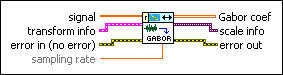signal specifies the input signal.transform info specifies the analysis window to use for the Gabor transform. You can use the Dual Window Express VI to design the analysis window.analysis window specifies the window to use to compute the Gabor coefficients. analysis window is the dual window of the synthesis window, which is the envelope of the Gabor elementary functions. The size of analysis window must be divided evenly by dM (time steps) and N (frequency bins).dM (time steps) specifies the time shift, in samples, between elementary functions. The time sampling interval of the signal in the time-frequency domain is dM (time steps)/fs, where fs is the sampling rate of the signal. National Instruments recommends that you set dM (time steps) to a power of 2.N (frequency bins) specifies the number of frequencies of the Gabor elementary functions. The frequency sampling interval of the signal in the time-frequency plane is fs/N (frequency bins), where fs is the sampling rate of the signal. N (frequency bins) must be a power of 2. For stable reconstruction, N (frequency bins) must be greater than or equal to dM (time steps). The ratio between N (frequency bins) and dM (time steps) is called the oversampling rate.error in describes error conditions that occur before this node runs. This input provides standard error in functionality.sampling rate specifies the sampling rate of signal in hertz. sampling rate must be greater than 0, or this VI sets sampling rate to 1 automatically. The default is 1.Gabor coef returns the linear time-frequency representation of the signal. Gabor coef contains the weights of the Gabor elementary functions. The element in the ith row and the jth column is the weight of the Gabor elementary function whose time center and frequency center equal i×dt and j×df, respectively, where dt is the time interval between every two contiguous rows of Gabor coef, and df is the frequency interval between every two contiguous columns of Gabor coef. You can use the TFA Coefficients Indicator to display the magnitude of Gabor coef on an intensity graph. You can save the time-dependent 2D array to a text file for use in another software environment. The resulting text file contains only Z values and does not retain the time axis information or the frequency axis information. You can use the TFA Get Time and Freq Scale Info VI to compute the time scale information and the frequency scale information of the time-frequency representation.scale info returns the time scale and the frequency scale information of the time-frequency representation, including the time offset, the time interval between every two contiguous rows, the frequency offset, and the frequency interval between every two contiguous columns of Gabor coef. Use the TFA Get Time and Freq Scale Info VI to return detailed information about the time scale and the frequency scale.error out contains error information. This output provides standard error out functionality.

## TFA Discrete Gabor Transform (Complex)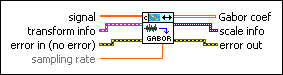signal specifies the input signal.transform info specifies the analysis window to use for the Gabor transform. You can use the Dual Window Express VI to design the analysis window.analysis window specifies the window to use to compute the Gabor coefficients. analysis window is the dual window of the synthesis window, which is the envelope of the Gabor elementary functions. The size of analysis window must be divided evenly by dM (time steps) and N (frequency bins).dM (time steps) specifies the time shift, in samples, between elementary functions. The time sampling interval of the signal in the time-frequency domain is dM (time steps)/fs, where fs is the sampling rate of the signal. National Instruments recommends that you set dM (time steps) to a power of 2.N (frequency bins) specifies the number of frequencies of the Gabor elementary functions. The frequency sampling interval of the signal in the time-frequency plane is fs/N (frequency bins), where fs is the sampling rate of the signal. N (frequency bins) must be a power of 2. For stable reconstruction, N (frequency bins) must be greater than or equal to dM (time steps). The ratio between N (frequency bins) and dM (time steps) is called the oversampling rate.error in describes error conditions that occur before this node runs. This input provides standard error in functionality.sampling rate specifies the sampling rate of signal in hertz. sampling rate must be greater than 0, or this VI sets sampling rate to 1 automatically. The default is 1.Gabor coef returns the linear time-frequency representation of the signal. Gabor coef contains the weights of the Gabor elementary functions. The element in the ith row and the jth column is the weight of the Gabor elementary function whose time center and frequency center equal i×dt and j×df, respectively, where dt is the time interval between every two contiguous rows of Gabor coef, and df is the frequency interval between every two contiguous columns of Gabor coef. You can use the TFA Coefficients Indicator to display the magnitude of Gabor coef on an intensity graph. You can save the time-dependent 2D array to a text file for use in another software environment. The resulting text file contains only Z values and does not retain the time axis information or the frequency axis information. You can use the TFA Get Time and Freq Scale Info VI to compute the time scale information and the frequency scale information of the time-frequency representation.scale info returns the time scale and the frequency scale information of the time-frequency representation, including the time offset, the time interval between every two contiguous rows, the frequency offset, and the frequency interval between every two contiguous columns of Gabor coef. Use the TFA Get Time and Freq Scale Info VI to return detailed information about the time scale and the frequency scale.error out contains error information. This output provides standard error out functionality.

## TFA Discrete Gabor Transform (2D)Note  Computes the separable 2D discrete Gabor transform.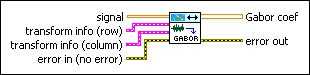signal specifies the 2D input signal.transform info (row) specifies the analysis window to use for the Gabor transform along each row of signal. You can use the Dual Window Express VI to design the analysis window.analysis window specifies the window to use to compute the Gabor coefficients. analysis window is the dual window of the synthesis window, which is the envelope of the Gabor elementary functions. The size of analysis window must be divided evenly by dM (time steps) and N (frequency bins).dM (time steps) specifies the time shift, in samples, between elementary functions. The time sampling interval of the signal in the time-frequency domain is dM (time steps)/fs, where fs is the sampling rate of the signal. National Instruments recommends that you set dM (time steps) to a power of 2.N (frequency bins) specifies the number of frequencies of the Gabor elementary functions. The frequency sampling interval of the signal in the time-frequency plane is fs/N (frequency bins), where fs is the sampling rate of the signal. N (frequency bins) must be a power of 2. For stable reconstruction, N (frequency bins) must be greater than or equal to dM (time steps). The ratio between N (frequency bins) and dM (time steps) is called the oversampling rate.transform info (column) specifies the analysis window to use for the Gabor transform along each column of signal. You can use the Dual Window Express VI to design the analysis window.analysis window specifies the window to use to compute the Gabor coefficients. analysis window is the dual window of the synthesis window, which is the envelope of the Gabor elementary functions. The size of analysis window must be divided evenly by dM (time steps) and N (frequency bins).dM (time steps) specifies the time shift, in samples, between elementary functions. The time sampling interval of the signal in the time-frequency domain is dM (time steps)/fs, where fs is the sampling rate of the signal. National Instruments recommends that you set dM (time steps) to a power of 2.N (frequency bins) specifies the number of frequencies of the Gabor elementary functions. The frequency sampling interval of the signal in the time-frequency plane is fs/N (frequency bins), where fs is the sampling rate of the signal. N (frequency bins) must be a power of 2. For stable reconstruction, N (frequency bins) must be greater than or equal to dM (time steps). The ratio between N (frequency bins) and dM (time steps) is called the oversampling rate.error in describes error conditions that occur before this node runs. This input provides standard error in functionality.Gabor coef returns the result of the 2D discrete Gabor transform.error out contains error information. This output provides standard error out functionality.

## TFA Discrete Gabor Transform Details

The Gabor elementary functions are localized in the joint time-frequency domain. The weight of the Gabor elementary function reveals the behavior of a signal around the time-frequency center of the Gabor elementary function in the joint time-frequency domain. The Gabor transform is a type of invertible linear transform.

## Examples

Refer to the following VIs for examples of using the TFA Discrete Gabor Transform VI:

• Gabor Transform and Expansion VI: labview\examples\Time Frequency Analysis\TFAFunctions
• Dual Function VI: labview\examples\Time Frequency Analysis\TFAGettingStarted
• Linear JTFA Method VI: labview\examples\Time Frequency Analysis\TFAGettingStarted